×#### Thank you for registering.

One of our academic counsellors will contact you within 1 working day.

Click to Chat

1800-1023-196

+91-120-4616500

CART 0

• 0

MY CART (5)

Use Coupon: CART20 and get 20% off on all online Study Material

ITEM
DETAILS
MRP
DISCOUNT
FINAL PRICE
Total Price: Rs.

There are no items in this cart.
Continue Shopping• Complete JEE Main/Advanced Course and Test Series
• OFFERED PRICE: Rs. 15,900
• View Details

```Chapter 8: Quadratic Equations Exercise – 8.6

Question: 1

Determine the nature of the roots of the following quadratic equations.

(i) 2x2 – 3x + 5 = 0

(ii) 2x2 - 6x + 3 = 0

(iii) For what value of k (4 - k)x2 + (2k + 4)x + (8k + 1) = 0 is a perfect square.

(iv) Find the least positive value of k for which the equation x2 + kx + 4 = 0 has real roots.

(v) Find the value of k for which the given quadratic equation has real roots and distinct roots.

Kx2 + 2x + 1 = 0

(vi) Kx2 + 6x + 1 = 0

(vii) x2 - kx + 9 = 0

Solution:

The given quadratic equation is in the form of ax2 + bx + c = 0

So a = 2, b = – 3, c = 5

We know, determinant (D) = b2 - 4ac = (-3)2 - 4(2)(5) = 9 - 40 = – 31 < 0

Since D < 0, the determinant of the equation is negative, so the expression does not having any real roots.

(ii) 2x2 - 6x + 3 = 0
The given quadratic equation is in the form of ax2 + bx + c = 0

So a = 2, b = -6, c = 3

We know, determinant (D) = b2 - 4ac = (- 6)2 - 4(2)(3) = 36 - 24 = 12 < 0

Since D > 0, the determinant of the equation is positive, so the expression does having any real and distinct roots

(iii) For what value of k (4 - k)x2 + (2k + 4)x + (8k + 1) = 0 is a perfect square.

The given equation is (4 - k)x2 + (2k + 4)x + (8k + 1) = 0

Here, a = 4 - k, b = 2k + 4, c = 8k + 1

The discriminate (D) = b2 - 4ac = (2k+4)2 - 4(4 - k)(8k + 1)

= (4k2 + 16 + 16k) - 4(32k + 4 - 8k2 - k)

= 4(k2 + 8k2 + 4k - 31k + 4-4)

= 4(9k2 - 27k)

D = 4(9k2 - 27k)

The given equation is a perfect square D = 0

4(9k2 - 27k) = 0

9k2 - 27k = 0

Taking out common of 3 from both sides and cross multiplying = k2 - 3k = 0

= K (k - 3) = 0

Either k = 0 Or k = 3

The value of k is to be 0 or 3 in order to be a perfect square.

(iv)Find the least positive value of k for which the equation x2 + kx + 4 = 0 has real roots.

The given equation is x2 + kx + 4 = 0 has real roots Here, a = 1, b = k, c = 4

The discriminate (D) = b2 - 4ac =  0 = k2 - 16 = 0 = k = 4, k = – 4

The least positive value of k = 4 for the given equation to have real roots.

(v) Find the value of k for which the given quadratic equation has real roots and distinct roots.
Kx2 + 2x + 1 = 0

The given equation is Kx2 + 2x + 1 = 0

Here, a = k, b = 2, c = 1

The discriminate (D) = b2 - 4ac = 0

= 4 - 4k = 0

= 4k = 4

K = 1

The value of k = 1 for which the quadratic equation is having real and equal roots.
(vi) Kx2 + 6x + 1 = 0

The given equation is Kx2 + 6x + 1 = 0

Here, a = k, b = 6, c = 1

The discriminate (D) = b2 - 4ac = 0

= 36 - 4k = 0

= 4k = 36

K = 9

The value of k = 9 for which the quadratic equation is having real and equal roots.

(vii) x2 - kx + 9 = 0

The given equation is x2 - kx + 9 = 0

Here, a = 1, b = – k, c = 9

Given that the equation is having real and distinct roots.

Hence, the discriminate (D) = b2 -  4ac = 0

= k2 - 4(1)(9) = 0

= k2 – 36 = 0

= K = – 6 and k = 6

The value of k lies between -6 and 6 respectively to have the real and distinct roots.

Question: 2

Find the value of k (i) Kx2 + 4x + 1 = 0.

(ii)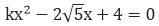(iii) 3x2 - 5x + 2k = 0

(iv) 4x2 + kx + 9 = 0

(v) 2kx2 - 40x + 25 = 0

(vi) 9x2 - 24x + k = 0

(vii) 4x2- 3kx + 1 = 0

(viii) x2 - 2(5 + 2k)x + 3(7 + 10k) = 0

(ix) (3k +1)x2+ 2(k +1)x + k = 0

(x) Kx2 + kx + 1 = – 4x2 - x

(xi) (k + 1)x2 + 2(k + 3)x + k + 8 = 0

(xii) x2 - 2kx + 7k - 12 = 0

(xiii) (k + 1)x2 - 2(3k + 1)x + 8k + 1 = 0

(xiv) 5x2 - 4x + 2 + k(4x2 - 2x + 1) = 0

(xv) (4 - k)x2 + (2k + 4)x + (8k + 1) = 0

(xvi) (2k + 1)x2 + 2(k + 3)x + (k +5 ) = 0

(xvii) 4x2 - 2(k + 1)x + (k + 4) = 0

Solution:

The given equation Kx2 + 4x + 1 = 0 is in the form of ax2 + bx + c = 0

Where a = k, b = 4, c = 1

Given that, the equation has real and equal roots D = b2 - 4ac = 0

= 42 - 4(k)(1) = 0

= 16 – 4k = 0

= k = 4

The value of k is 4

(ii)The given equationis in the form of ax2 + bx + c = 0 where a= k, b = - 2√5, c = 4.

Given that, the equation has real and equal roots D = b2-  4ac = 020 - 16k = 0

K = 5 /4

The value of k is k = 5/4

(iii) 3x2 - 5x + 2k = 0

The given equation 3x2 - 5x + 2k = 0 is in the form of ax2 + bx + c = 0 where a = 3, b = – 5, c = 2k

Given that, the equation has real and equal roots D = b2 - 4ac = 0

= (- 5)2 - 4(3)(2k) = 0

= 25 - 24k = 0

K = 25/24

The value of the k is k = 25/24

(iv) 4x2 + kx + 9 = 0

The given equation 4x2 + kx + 9 = 0 is in the form of ax2 + bx + c = 0 where a = 4, b = k, c = 9

Given that, the equation has real and equal roots D = b2 - 4ac = 0

= k2 - 4(4)(9) = 0

= k2 - 144 = 0

= k = 12

The value of k is 12

(v) 2kx2 - 40x + 25 = 0

The given equation 2kx2 - 40x + 25 = 0 is in the form of ax2 + bx + c = 0 where a = 2k, b = – 40, c = 25

Given that, the equation has real and equal roots D = b2 - 4ac = 0

(-40)2 - 4(2k)(25) = 0

1600 - 200k = 0

k = 8

The value of k is 8

(vi) 9x2 - 24x + k = 0

The given equation 9x2 - 24x + k = 0 is in the form of ax2 + bx + c = 0 where a = 9, b = – 24, c = k

Given that, the equation has real and equal roots D = b2 - 4ac = 0

( – 24)2 - 4(9)(k) = 0

576 - 36k = 0

k = 16

The value of k is 16

(vii) 4x2- 3kx + 1 = 0

The given equation 4x2 - 3kx + 1 = 0 is in the form of ax2 + bx + c = 0 where a = 4, b = - 3k, c = 1

Given that, the equation has real and equal roots D = b2 - 4ac = 0

= (-3k)2 - 4(4)(1) = 0

= 9k2 - 16 = 0

K = 4/3

The value of k is 4/3

(viii) x2 - 2(5 + 2k)x + 3(7 + 10k) = 0

The given equation X2 - 2(5 + 2k)x + 3(7 + 10k) = 0 is in the form of ax2 + bx + c = 0 where a = 1, b = +2(52k), c = 3(7 + 10k)

Given that, the nature of the roots of the equation are real and equal roots D = b2 - 4ac = 0

= (+2(52k))2 - 4(1)(3(7 + 10k)) = 0

= 4(5 + 2k)2 - 12(7 + 10k) = 0

= 25 + 4k2 + 20k - 21 - 30k = 0

= 4k2 - 10k + 4 = 0

Simplifying the above equation.

We get, = 2k2 - 5k + 2 = 0

= 2k2 - 4k - k + 2 = 0

= 2k(k - 2) - 1(k - 2) = 0

= (k - 2)(2k - 1) = 0, K = 2 and k = 1/2 The value of k can either be 2 or 1/2
(ix) (3k +1)x2+ 2(k +1)x + k = 0

The given equation (3k + 1)x2 + 2(k + 1)x + k = 0 is in the form of ax2 + bx + c = 0 where a = 3k + 1, b = +2(k + 1), c = (k)

Given that, the nature of the roots of the equation are real and equal roots D = b2 - 4ac = 0

= [2(k + 1)]2 - 4(3k + 1)(k) = 0

= (k + 1)2 - k(3k + 1) = 0

= -2k2 + k + 1 = 0

This equation can also be written as 2k2 - k - 1 = 0

The value of k can be obtained by k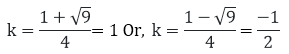The value of k are 1 and (-1)/2 respectively.
(x) Kx2 + kx + 1 = -4x2 - x

Bringing all the x components on one side we get, x2(4 + k) + x(k + 1) + 1 = 0

The given equation Kx2 + kx + 1 = -4x2 - x is in the form of ax2 + bx + c = 0 where a = 4 + k,b = +k + 1, c = 1 Given that, the nature of the roots of the equation are real and equal roots D= b2- 4ac = 0

= (k+1)2- 4(4 + k)(1) = 0

= k2- 2k – 10 = 0

The equation is also in the form ax2 + bx + c = 0

The value of k is obtained by a = 1, b = -2, c = – 15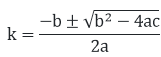Putting the respective values in the above formula we will obtain the value of k

The value of k are 5 and -3 for different given quadratic equation.

(xi) (k + 1)x2 + 2(k + 3)x + k + 8 = 0

The given equation (k + 1) x2 + 2(k + 3)x + k + 8 = 0 is in the form of ax2 + bx + c = 0 where a = k + 1,b = 2(k + 3), c = k + 8

Given the nature of the roots of the equation are real and equal. D = b2 - 4ac = 0

= [2(k + 30]2 - 4(k + 1)(k + 8) = 0

= 4(k + 3)2 - 4(k + 1)(k + 8) = 0

Taking out 4 as common from the LHS of the equation and dividing the same on the RHS = (k + 3)2-(k + 1)(k + 8) = 0

= k2 + 9 + 6k - (k2 + 9k + 18) = 0

Cancelling out the like terms on the LHS side = 9 + 6k - 9k - 8 = 0

= - 3k + 1 = 0

= 3k = 1

K = 1/3

The value of k of the given equation is k =1/3

(xii) x2 - 2kx + 7k - 12 = 0

The given equation is x2 - 2kx + 7k - 12 = 0

The given equation is in the form of ax2 + bx + c = 0 where a = 1, b = – 2k, c = 7k – 12

Given the nature of the roots of the equation are real and equal. D = b2 - 4ac = 0

= (2k)2 - 4(1)(7k - 12) = 0

= 4k2 - 28k + 48 = 0

= k2 - 7k + 12 = 0

The value of k can be obtained by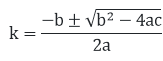Here a = 1, b = – 7k, c = 12

By calculating the value of k is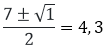The value of k for the given equation is 4 and 3 respectively.

(xiii) (k + 1)x2 - 2(3k + 1)x + 8k + 1 = 0

The given equation is (k + 1)x2 - 2(3k + 1)x + 8k + 1 = 0

The given equation is in the form of ax2 + bx + c = 0 where a = k + 1, b = – 2(k + 1), c = 8k + 1

Given the nature of the roots of the equation are real and equal. D = b2- 4ac = 0

= (-2(k + 1))2 - 4(k + 1)(8k + 1) = 0

= 4(3k + 1)2 - 4(k + 1)(8k + 1) = 0

Taking out 4 as common from the LHS of the equation and dividing the same on the

RHS = (3k + 1)2 - (k + 1)(8k + 1) = 0

= 9k2 + 6k + 1 – (8k2 + 9k + 1) = 0

= 9k2 + 6k + 1 – 8k2 - 9k - 1 = 0

= k2 - 3k = 0

= k(k - 3) = 0

Either k = 0 Or, k - 3 = 0 = k = 3

The value of k for the given equation is 0 and 3 respectively.

(xiv) 5x2 - 4x + 2 + k(4x2 - 2x + 1) = 0

The given equation 5x2 - 4x + 2 + k(4x2 - 2x + 1) = 0 can be written as x2(5 + 4k) - x(4 + 2k) + 2 - k = 0

The given equation is in the form of ax2 + bx + c = 0 where a = 5 + 4k, b = -(4 + 2k), c = 2 - k

Given the nature of the roots of the equation are real and equal.

D = b2 - 4ac = 0

= [-(4 + 2k)]2 - 4(5 + 4k)(2 - k) = 0

= 16 + 4k2 + 16 - 4(10 - 5k + 8k - 4k2] = 0

= 16 + 4k2 + 16 - 40 + 20k - 32k + 16k2 = 0

= 20k2 - 4k - 24 = 0

Taking out 4 as common from the LHS of the equation and dividing the same on the RHS = 5k2 - k - 6 = 0 The value of k can be obtained by equation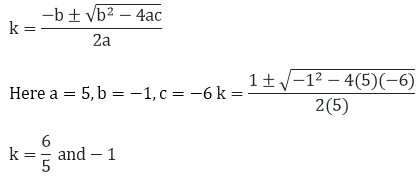The value of k for the given equation are k = 6/5 and−1 respectively.

(xv) (4 - k)x2 + (2k + 4)x + (8k + 1) = 0

The given equation is (4 - k)x2 + (2k + 4)x + (8k + 1) = 0

The given equation is in the form of ax2 + bx + c = 0 where a = 4 - k, b = (2k + 4), c = 8k + 1

Given the nature of the roots of the equation are real and equal.

D = b2 - 4ac = 0

= (2k + 4)2 - 4(4 - k)(8k + 1) = 0

= 4k2 + 16k + 16 - 4(-8k2 + 32k + 4 - k) = 0

= 4k2 + 16k + 16 + 32k2 - 124k - 16 = 0

Cancelling out the like and opposite terms.

We get, = 36k2 - 108k = 0

Taking out 4 as common from the LHS of the equation and dividing the same on the RHS = 9k2 - 27k = 0

= 9k(k -3 ) = 0

Either 9k = 0 K = 0 Or, k - 3 = 0 K = 3

The value of k for the given equation is 0 and 3 respectively.

(xvi) (2k + 1)x2 + 2(k + 3)x + (k +5 )= 0

The given equation is (2k + 1)x2 + 2(k + 3)x + (k + 5)  = 0

The given equation is in the form of ax2 + bx + c = 0 where a = 2k + 1, b = 2(k + 3), c = k + 5

Given the nature of the roots of the equation are real and equal.

D = b2 - 4ac = 0

= [2(k + 3)]2 - 4(2k + 1)(k + 5) = 0

Taking out 4 as common from the LHS of the equation and dividing the same on the

RHS = [(k + 3)]2 - (2k + 1)(k + 5) = 0

= K2 + 9 + 6k - (2k2 + 11k + 5) = 0

= – k2 - 5k + 4 = 0

= k2 + 5k - 4 = 0

The value of k can be obtained by k = 6/5 and − 1 respectively.

Here a = 1, b = 5, c = – 4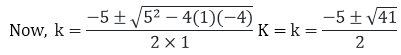The value of k for the given equation is(xvii) 4x2 - 2(k + 1)x + (k + 4) = 0

The given equation is 4x2 - 2(k + 1)x + (k + 4) = 0

The given equation is in the form of ax2 + bx + c = 0 where a = 4, b = -2(k + 1), c = k + 4

Given the nature of the roots of the equation are real and equal.

D = b2 - 4ac = 0

= [-2(k + 1)]2 - 4(4)(k + 4) = 0

Taking out 4 as common from the LHS of the equation and dividing the same on the

RHS = (k + 1)2 - 4(k + 4) = 0

= k2 + 1 + 2k - 4k - 16 = 0

= k2 - 2k - 15 = 0

The value of k can be obtained by k = 6/5 and − 1 respectively.

Here a = 1, b = -2, c = -15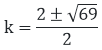The value of k for the given equation isQuestion: 3

In the following, determine the set of values of k for which the given quadratic equation has real roots:

(i) 2x2 + 3x + k = 0

(ii) 2x2 + kx + 3 = 0

(iii) 2x2 - 5x - k = 0

(iv) Kx2 + 6x + 1= 0

(v) x2 - kx + 9 = 0

Solution:

(i) 2x2 + 3x + k = 0

The given equation is 2x2 + 3x +k = 0

The given quadratic equation has equal and real roots D = b2- 4ac = 0

The given equation is in the form of ax2 + bx + c = 0 so, a = 2, b = 3, c = k = 9 - 4(2)(k) = 0

= 9 - 8k = 0

= k ≤ 98

The value of k does not exceed k ≤ 98 to have a real root.

(ii) 2x2 + kx + 3 = 0

The given equation is 2x2 + kx + 3 = 0

The given quadratic equation has equal and real roots D = b2 - 4ac = 0

The given equation is in the form of ax2 + bx + c = 0 so, a = 2, b = k, c = 3 = k2 - 4(2)(3) = 0

= k2 – 24 = 0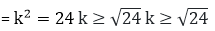The value of k should not exceedin order to obtain real roots.

(iii) 2x2 - 5x - k = 0

The given equation is 2x2 - 5x - k = 0

The given quadratic equation has equal and real roots D = b2 - 4ac = 0

The given equation is in the form of ax2 + bx + c = 0 so, a = 2, b = - 5, c = - k = 25 - 4(2)(- k) = 0

= 25 - 8k = 0

= k ≤ 25/8

The value of k should not exceed k ≤ 25/8
(iv) Kx2 + 6x + 1= 0

The given equation is Kx2 + 6x + 1 = 0

The given quadratic equation has equal and real roots D = b2 - 4ac = 0

The given equation is in the form of ax2 + bx + c = 0 so, a = k, b = 6, c = 1 = 36 - 4(k)(1) = 0

= 36 - 4k = 0

= k = 9

The value of k for the given equation is k = 9

(v) x2 - kx + 9 = 0

The given equation is X2 - kx + 9 = 0

The given quadratic equation has equal and real roots D = b2- 4ac = 0

The given equation is in the form of ax2 + bx + c = 0 so, a = 1, b = -k, c = 9 = k2 - 4(1)(-9) = 0

= k2 – 36 = 0

= k2 = 36 k ≥ √36 K = 6 and k = - 6

The value of k should in between K = 6 and k = - 6 in order to maintain real roots.

Question: 4

Determine the nature of the roots of the following quadratic equations.

(i) 2x2 - 3x + 5 = 0

(ii) 2x2 - 6x + 3 = 0

(iii) For what value of k (4 - k)x2 + (2k + 4)x + (8k + 1) = 0 is a perfect square

(iv) Find the least positive value of k for which the equation x2 + kx + 4 = 0 has real roots.

(v) Find the value of k for which the given quadratic equation has real roots and distinct roots.

Kx2 + 2x + 1 = 0

(vi) Kx2 + 6x + 1 = 0

(vii) x2  – kx + 9 = 0

Solution:

(i) 2x2 - 3x + 5 = 0

The given quadratic equation is in the form of ax2 + bx + c = 0

So a = 2, b = -3, c = 5

We know, determinant (D) = b2 – 4ac = (-3)2 – 4(2)(5)

= 9 – 40 = – 31 < 0

Since D < 0, the determinant of the equation is negative, so the expression does not having any real roots.

(ii) 2x2 - 6x + 3 = 0

The given quadratic equation is in the form of ax2 + bx + c = 0

So a = 2, b = -6, c = 3

We know, determinant (D) = b2 – 4ac = (-6)2 - 4(2)(3) = 36 – 24 = 12 < 0

Since D > 0, the determinant of the equation is positive, so the expression does having any real and distinct roots.
(iii) For what value of k (4 - k)x2 + (2k + 4)x + (8k + 1) = 0 is a perfect square

The given equation is (4 - k)x2 + (2k + 4)x + (8k + 1) = 0

Here, a = 4 - k, b = 2k + 4, c = 8k + 1

The discriminate (D) = b2 – 4ac

= (2k + 4)2 – 4(4 - k)(8k + 1)

= (4k2 + 16 + 16k) - 4(32k + 4 - 8k2 - k)

= 4(k2 + 8k2 + 4k - 31k + 4 - 4)

= 4(9k2 - 27k)

= 4(9k2 - 27k)

The given equation is a perfect square D = 0

4(9k2 - 27k) = 0

9k2 - 27k = 0

Taking out common of 3 from both sides and cross multiplying K2 - 3k = 0 K (k - 3) = 0

Either k = 0 Or k = 3

The value of k is to be 0 or 3 in order to be a perfect square.

(iv) Find the least positive value of k for which the equation x2 + kx + 4 = 0 has real roots.

The given equation is x2 + kx + 4 = 0 has real roots Here, a = 1, b = k, c = 4

The discriminate (D) = b2 – 4ac = 0

= k2 – 16 = 0

= k = 4, k = - 4

The least positive value of k = 4 for the given equation to have real roots.

(v) Find the value of k for which the given quadratic equation has real roots and distinct roots.

Kx2 + 2x + 1 = 0

The given equation is Kx2 + 2x + 1 = 0

Here, a = k, b = 2, c = 1

The discriminate (D) = b2 – 4ac = 0

= 4 - 4k = 0

= 4k = 4

K = 1

The value of k = 1 for which the quadratic equation is having real and equal roots.

(vi) Kx2 + 6x + 1 = 0

The given equation is Kx2 + 6x + 1 = 0

Here, a = k, b = 6, c = 1

The discriminate (D) = b2 – 4ac = 0

= 36 - 4k = 0

= 4k = 36

= K = 9

The value of k = 9 for which the quadratic equation is having real and equal roots.

(vii) x2  – kx + 9 = 0

The given equation is X2 – kx + 9 = 0

Here, a = 1, b = -k, c = 9

Given that the equation is having real and distinct roots. Hence, the discriminate (D) = b2 – 4ac = 0

= k2 – 4(1)(9) = 0

= k2 - 36 = 0

= k = – 6 and k = 6

The value of k lies between -6 and 6 respectively to have the real and distinct roots.

Question: 5

Find the values of k for which the given quadratic equation has real and distinct roots.

(i) Kx2 + 2x + 1 = 0

(ii) Kx2 + 6x + 1 = 0

Solution:

(i) Kx2 + 2x + 1 = 0

The given equation is Kx2 + 2x + 1 = 0

The given equation is in the form of ax2 + bx + c = 0 so, a = k, b = 2, c = 1 D = b2 - 4ac = 0

= 4 - 4(1)(k) = 0

= 4k = 4

k = 1

The value of k for the given equation is k = 1
(ii) Kx2 + 6x + 1 = 0

The given equation is Kx2 + 6x + 1 = 0

The given equation is in the form of ax2 + bx + c = 0 so, a = k, b = 6, c = 1

D = b2 - 4ac = 0

= 36 - 4(1)(k) = 0

= 4k = 36

= k = 9

The value of k for the given equation is k = 9

Question: 6

For what value of k, (4 - k)x2 + (2k + 4)x + (8k + 1) = 0, is a perfect square.

Solution:

The given equation is (4 - k)x2 + (2k + 4)x + (8k + 1) = 0

The given equation is in the form of ax2 + bx + c = 0 so, a = 4 - k, b = 2k + 4, c = 8k + 1 D = b2 - 4ac

= (2k + 4)2 - 4(4 - k)(8k + 1)

= 4k2 + 16 + 4k - 4(32 + 4 - 8k2 - k)

= 4(k2 + 4 + k - 32 - 4 + 8k2 + k)

=4(9k2 - 27k)

Since the given equation is a perfect square Therefore D = 0 = 4(9k2 - 27k) = 0

= (9k2 - 27k) = 0

= 3k(k - 3) = 0

Therefore 3k = 0

K = 0 Or, k-3 = 0 K = 3 The value of k should be 0 or 3 to be perfect square.

Question: 7

If the roots of the equation (b - c)x2 + (c - a)x + (a - b) = 0 are equal , then prove that 2b = a + c.

Solution:

The given equation is (b - c)x2 + (c - a)x + (a - b) = 0.

The given equation is the form of ax2 + bx + c = 0.

So, a = (b - c), b = (c - a), c = (a - b)

According to question the equation is having real and equal roots.

Hence discriminant (D) = b2 - 2ac = 0

= (c - a)2 - 4(b - c)(a - b) = 0

= c2 + a2 - 2ac – 4(ab - b2 - ac + cb) = 0

= c2 + a2 - 2ac – 4ab + 4b2 + 4ac - 4cb = 0

= c2 + a2 + 2ac – 4ab + 4b2 - 4cb = 0

= (a + c)2 - 4ab + 4b2 - 4cb = 0

= (c + a - 2b)2 = 0

= (c + a - 2b) = 0

= c + a = 2b

Hence it is proved that c + a = 2b.

Question: 8

If the roots of the equation (a2 + b2) x2 – 2(ac + bd)x + (c2 + d2) = 0 are equal. Prove that a ÷ b = c ÷ d.

Solution:

The given equation is (a2 + b2)x2 – 2(ac + bd)x + (c2 + d2) = 0.

The equation is in the form of ax2 + bx = c = 0

Hence, a = (a2 + b2), b = – 2(ac + bd), c = (c2 + d2).

The given equation is having real and equal roots.

Discriminant (D) = b2 - 4ac = 0

= [-2(ac + bd)]2 - 4 (a2 +b2)(c2 + d2) = 0

= (ac + bd)2 - (a2 + b2)(c2 + d2) = 0

= a2c2 + b2d2 + 2abcd – (a2c2 + a2d2 + b2c2 + b2d2) = 0

Cancelling out the equal and opposite terms.

We get, = 2abcd - a2d2 - b2c2 = 0

= abcd + abcd - a2d2 - b2c2 = 0

= ad – bc = 0

= (a ÷ b) = (c ÷ d)

Hence, it is proved.

Question: 9

If the roots of the equation ax2 + 2bx + c = 0 and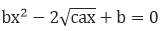are simultaneously real, then prove that b2 - ac = 0.

Solution:

The given equations are ax2 + 2bx +c = 0 andThese two equations are of the form ax2 + bx + c = 0.

Given that the roots of the two equations are real.

Hence, D = 0 that is b2 - 4ac = 0

Let us assume that ax2 + 2bx + c = 0 be equation (i) andbe (ii) From equation (i) b2 - 4ac = 0

= 4 b2 - 4ac = 0  .... (iii)

From equation (ii) b2 - 4ac = 0Given, that the roots of equation (i) and (ii) are simultaneously real and hence equation (iii) = equation (iv).

= 4b2 - 4ac = 4ac - 4

b2 = 8ac = 8b2 = b2 - ac = 0.

Hence it is proved that b2 - ac = 0.

Question: 10

If p, q are the real roots and p = q. Then show that the roots of the equation (p-q)x2 + 5(p + q)x - 2(p - q) = 0 are real and equal.

Solution:

The given equation is (p - q)x2 + 5(p + q)x - 2(p - q) = 0

Given, p , q are real and p ? q.

Then, Discriminant (D) = b2– ac = [5(p + q)]2 - 4(p - q)(-2(p - q)) = 25(p + q)2 + (p - q)2

We know that the square of any integer is always positive that is, greater than zero.

Hence, (D) = b2 –ac = 0 As given, p, q are real and p = q.

Therefore, = 25(p + q)2 + (p - q)2 ? 0 = D = 0

Therefore, the roots of this equation are real and unequal.

Question: 11

If the roots of the equation (c2 - ab)x2 - 2(a2 - bc)x + b2 - ac = 0 are equal , then prove that either a = 0 or a³ + b³ + c³ = 3abc .

Solution:

The given equation is (c2 - ab)x2 - 2(a2 - bc)x + b2 - ac = 0

This equation is in the form of ax2  + bx + c = 0

So, a = (c2 - ab), b = -2(a2 - bc), c = b2 - ac.

According to the question, the roots of the given question are equal.

Hence, D= 0, b2 - 4ac = 0

= [-2(a2 - bc)]2 - 4(c2 - ab)( b2 - ac) = 0

= 4(a2 - bc)2 - 4(c2 - ab)( b2 - ac) = 0

= 4a(a³ + b³ + c³ - 3abc) = 0

Either 4a =0 therefore, a = 0 Or, (a³ + b³ + c³ - 3abc) = 0

= (a³ + b³ + c³) = 3abc Hence its is proved.

Question: 12

Show that the equation 2(a2 + b2)x2 + 2(a + b)x + 1 = 0 has no real roots , when a = b.

Solution:

The given equation is 2(a2 + b2)x2 + 2(a + b)x - 1 = 0

This equation is in the form of ax2 + bx + c = 0

Here, a = 2(a2 + b2), b = 2(a + b), c = +1.

Given, a = b The discriminant (D) = b2  - 4ac = [2(a + b)]2 - 4(2(a2 + b2))(1)

= 4(a + b)2 - 8(a2 + b2)

= 4(a2 + b2 + 2ab) – 8a2 - 8b2

= +2ab – 4a2 - 4b2

According to the question a = b, as the discriminant D has negative squares so the value of D will be less than zero. Hence, D = 0, when a = b.

Question: 13

Prove that both of the roots of the equation (x - a)(x - b) +(x - c)(x - b) + (x - c)(x - a) = 0 are real but they are equal only when a = b = c.

Solution:

The given equation is (x - a)(x - b) + (x - c)(x - b) + (x - c)(x - a) = 0

By solving the equation, we get it as, 3x2 - 2x(a + b + c) + (ab + bc + ca) = 0

This equation is in the form of ax2 + bx + c = 0

Here, a = 3, b = 2(a + b + c), c = (ab + bc + ca)

The discriminate (D) =  b2 - 4ac

= [-2(a + b + c)]2 - 4(3)(ab + bc + ca)

= 4(a + b +c )2 -12(ab + bc + ca)

= 4[(a + b + c)2 - 3(ab + bc + ca)]

= 4[a2 + b2 + c2 – ab - bc - ca]

= 2[2a2 + 2b2 + 2c2 – 2ab - 2bc - 2ca]

= 2[(a - b)2 + (b - c)2 + (c - a)2]

Here clearly D = 0, if D = 0 then, [(a - b)2 + (b - c)2 + (c - a)2] = 0

a –b = 0 b – c = 0 c – a = 0

Hence, a = b = c = 0 Hence, it is proved.

Question: 14

If a, b, c are real numbers such that ac = 0, then, show that at least one of the equations ax2 + bx + c = 0 and – ax2 + bx + c = 0 has real roots.

Solution:

The given equation are ax2 + bx + c = 0 … (i)

And - ax2 + bx + c = 0 … (ii)

Given, equations are in the form of ax2+ bx + c = 0 also given that a, b, c are real numbers and ac = 0.

The Discriminant (D) = b2 - 4ac For equation (i) = b2 - 4ac ... (iii)

For equation (ii) = b2 - 4(-a)(c) = b2 + 4ac … (iv)

As a, b, c are real and given that ac = 0

Hence  b2 - 4ac = 0 and b2+ 4ac = 0 Therefore, D = 0 Hence proved.

Question: 15

If the equation (1 + m2)x + 2mcx + (c2 - a2) = 0 has real and equal roots , prove that c2 = a2(1 + m2).

Solution:

The given equation is (1 + m2)x2 + 2mcx + (c2 - a2) = 0

The above equation is in the form of ax2 + bx + c = 0.

Here a = (1 + m2), b = 2mc, c = +(c2 - a2)

Given, that the nature of the roots of this equation is equal and hence D = 0, b2 - 4ac = 0

= (2mc)2 - 4(1 + m2)(c2 - a2) = 0

= 4m2c2 – 4(c2 + m2c2 - a2 – a2m2) = 0

= 4(m2c2 - c2 + m2c2 + a2 + a2m2) = 0

= m2c2 - c2 + m2c2 + a2 + a2m2 = 0

Now cancelling out the equal and opposite terms, = a2 + a2m2 - c2 = 0

= a2 (1 + m2) – c2 = 0

Therefore, c2 = a2 (1 + m2) Hence it is proved that as D = 0, then the roots are equal of c2 = a2 (1+ m2).
```### Course Features

• 728 Video Lectures
• Revision Notes
• Previous Year Papers
• Mind Map
• Study Planner
• NCERT Solutions
• Discussion Forum
• Test paper with Video Solution